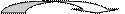Writing Expressions

The most important part of writing expressions is to know that words for addition, subtraction, multiplication and division. It is also important to know "turn around" words and words that indicate the use of parenthesis.

Sum

And

Plus

More than

Increased by

SUBTRACTION

Difference

Minus

Less

Fewer

Decreased by

"Turn around words"Less than

Fewer than

From

MULTIPLICATION

Product

Times

Of

Twice

Triple

DIVISION

Quotient

Per

Divided by

Ratio

(Parenthesis words)

Times the sum

Times the difference

Plus the difference of

Equal

Is

Total

The same as

Let's look at some examples of how to write expressions given a verbal description.

• The product of a number and 8    8n

• The sum of a number and 12    n + 12

• The quotient of a number and 8    $\frac{n}{8}$

• 12 increased by a number n    12 + n

• 15 less than a number    n - 15

• 4 times the sum of x and 3   4(x + 3)

• 12 miles per n gallons   12 miles/ n gallons

• n decreased by x    n - x

• y doubled   2y

• 5 less y    5 - y

• 5 less than y    y - 5

• 4 divided by y    $\frac{4}{y}$

• 4 divided into y    $\frac{y}{4}$

A little harder...

• five less than the product of 2 and x    2x - 5

• the difference of x and 3 divided by 6    $\frac{x-3}{6}$

• two less than one-fourth of y   1/4 y - 2

• fifteen decreased by the quotient of a number and 3    $15-\frac{n}{3}$

• the sum of twice a number and 5   2n + 5

• 12 more than the quotient of a number    $12+\frac{n}{5}=-10$

and 5 is negative 10

A little more challenging...

• Jim's age is 3 times Sarah's age   J = 3S

• The length is 5 more than the width   L = 5 + w

• The amount of money you will earn if you make $7 per hour for x hours A =$7x

• One third of a number increased by seven is five less than twice the number    1/3n +7 = 2n - 5

A few last tips...

Memorize the most common words for each operation. Always look for "turn around" and (parenthesis) words. When writing an expression for a word problem use variables that are representative of the problem. For example, lett h represent hours.

 Related Links: Math algebra Dependent and Independent Variables Measures of Variability : Mean Absolute Deviation

To link to this Writing Expressions page, copy the following code to your site: